Publication date: 07/30/2020

##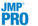Formulas for Metrics

The formulas for Coverage and Diversity depend on whether there are constraints. The following notation is used:

uCv is the number of combinations of u things taken v at a time

t is the strength of the design

K is the number of factors

M = KCt

i = 1, 2,..., M is an index that orders all combinations, or projections, of t factors

vik is the number of levels for the kth factor

ni is the number of distinct t tuples in the design for the ith projection

pi is the product of the vik for the factors in the ith projection

r is the number of runs in the design

###Unconstrained Design

Coverage and Diversity are given by the following: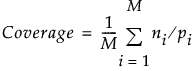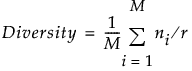###Constrained Design

In a constrained design, certain t tuples are not allowed. This can result in missing values for some t tuples. For some combinations of t factors, there might be no valid t tuples whatsoever. Coverage and diversity must be defined in terms of the possible valid combinations. For this reason, the formulas for constrained designs require additional notation:

ai is the number of invalid t tuples arising from factors in the ith projection

m is the number of projections where there are no valid t tuples

qi is the number of runs in the design with missing values for any factor in the ith projection

ri = r - qi

M = M - m

Coverage and Diversity are given by the following: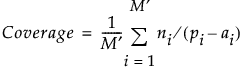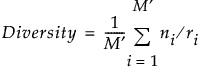If there are no invalid t tuples (M = M) and if there are no missing values (ri = r, for all i), then the definitions for coverage and diversity for constrained designs reduce to the definitions for unconstrained designs. See Morgan (2014).